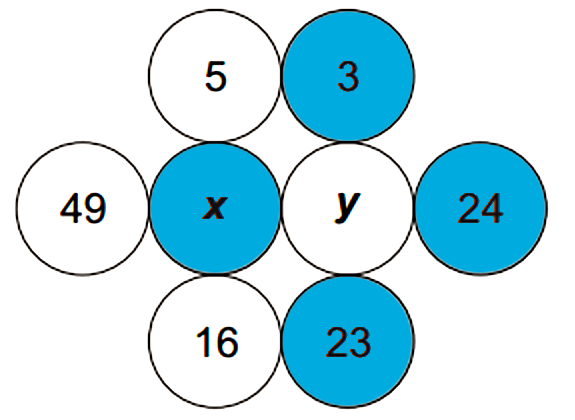# AM Circles

Probability Level 2The value of $x$ is the mean of the numbers in the white circles. The values of $y$ is the mean of numbers in the blue circles.

Find $x-y$.

×